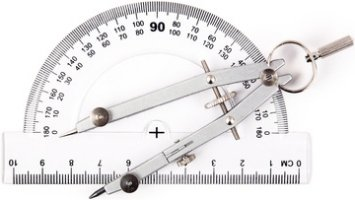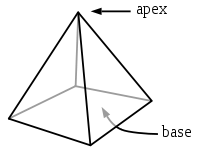## Angle conversionAngles are used in geometry, trigonometry, geography, topography and astronomy to measure space between 2 crossing lines (secants).

This angle converter will allow you to simultaneously convert plane angles between common units.

Geographic coordinates of the Global Positionning System (GPS) are expressed in the sexagesimal system (WGS84).
For example for the Greenwich latitude is 51° 28' 59'' 671 North or 51.4832419444° North (longitude is 0° west)

 degree it is the plane angle the most widely used. Protractors are usually scaled in degrees as in the picture radian is the official SI plane angle unit grade or gon whole turn 360° 2π rd (π, spelt "Pi", is the ratio of a circle's circumference to its diameter, π=3.1415926535...) 400 gon straight line 180° π rd 200 gon right angle 90° π/2 rd 100 gon

Enter the angle in the second column of the following array and the conversion will be performed automatically in all other units.

 Sexagesimal coordinates as in the GPS geodesic system Degree ° Minute of arc ' arcsecond '' thousandth of ''
degree °
turn/cycle/circle tr
military thousandth OTAN mil
Warsaw pact thousandth
thousandth (Sweden)
point
hour (out of 24)
hour (out of 12)
minute of arc '
arcsecond ''
cosinus cos
sinus sin
tangent tan
The selected angle is displayed in green on the following protractor picture :### Angle conversion of solid angle of a cone to an apex plane angleA solid angle is similar in 3 dimensions to a plane angle in 2 dimensions.
A solid angle Ω is equal to the ratio of the viewed surface A divided by the square of the viewed distance r.
Ω=A/r^2
It is expressed in steradian (sr), the official SI unit - international system of units. It is comprised of numbers between 0 and 4π sr for a whole sphere.

For a regular cones, solid angle Ω is equal to Ω =2 π x (1 - cos(θ/2))
where θ is the plane angle of a cone apex. For example, a hemisphère (half ball) has a plan angle θ of π rd and a solid angle of 2π sr
If we reverse the previous formula, we can deduct the plane angle θ from the solid angle Ω :
θ = 2 x arccos(1- Ω/2π)
For example, the apparent diameter of the moon seen from earth is θ=0.5 °, which is equivalent to a solid angle of about 6e-5 steradian.
Reading eyes vision field is θ=3 ° (0.002 sr) (foveal zone).
The peripheral vision field of eyes is about θ=25 ° (ellipse shape with -15° left, +15 ° right, -8° high, +12° low) so about 0.15 sr.

The following field is a solid angle Ω of a regular cone whose plane angle θ is indicated on the top array.